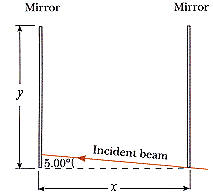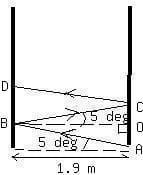Question# How many times will the incident beam shown in Figure (x = 1.90 m, y =1.00 m) be reflected by each of the parallel mirrors?FSP0251032571.gifFSZ

Other
ANSWEREDHow many times will the incident beam shown in Figure (x = 1.90 m, y =1.00 m) be reflected by each of the parallel mirrors?In the figure $$\displaystyle{A}{O}={O}{B}{{\tan{{5}}}^{\circ}}$$
$$\displaystyle{A}{C}={2}{O}{B}{{\tan{{5}}}^{\circ}=}{2}\times{\left({1.9}\ {m}\right)}\times{\left({0.087}\right)}={0.332}\ {m}$$